# MathML Browser Test (Presentation Markup)

This is an HTML5 using MathML document.
Click on a formula/equation to see the source code that generated it.

Formula Image of TeX rendering
(MiKTeX 2.9)
Image of MathML rendering with Firefox 84.0
using Latin Modern Math and STIX fonts
MathML rendering
(by this browser)
Axiom of
power set$∀ A ∃ P ∀ B [ B ∈ P ⟺ ∀ C ( C ∈ B ⇒ C ∈ A ) ]$
De Morgan's law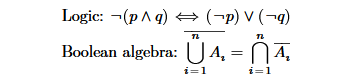Formula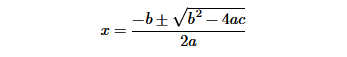$x = − b ± b 2 − 4 ⁢ a ⁢ c 2 ⁢ a$
Binomial
Coefficient$C ( n , k ) = C k n = C k n = ( n k ) = n ! k ! ⁢ ( n − k ) !$
Sophomore's
dream$∫ 0 1 x x ⁢ ⅆ x = ∑ n = 1 ∞ ( − 1 ) n + 1 ⁢ n − n$
Divergence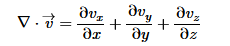$∇ · v → = ∂ v x ∂ x + ∂ v y ∂ y + ∂ v z ∂ z$
Complex
number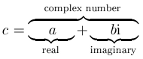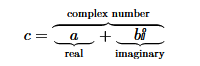$c = a ⏟ real + b ⁢ ⅈ ⏟ imaginary ⏞ complex number$
Moore
determinant$M = [ α 1 α 1 q … α 1 q n − 1 α 2 α 2 q … α 2 q n − 1 ⋮ ⋮ ⋱ ⋮ α m α m q … α m q n − 1 ]$
Sphere
volume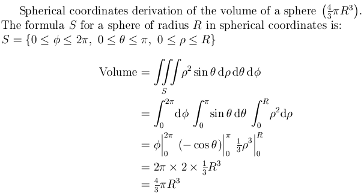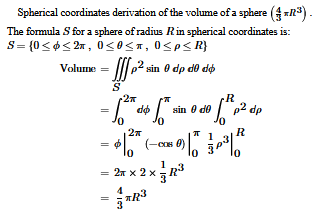Spherical coordinates derivation of the volume of a sphere $( 4 3 ⁢ π R 3 )$ .
The formula $S$ for a sphere of radius $R$ in spherical coordinates is:
$Volume = ∭ S ρ 2 ⁢ sin ⁡ θ ⁢ ⅆ ρ ⁢ ⅆ θ ⁢ ⅆ ϕ = ∫ 0 2 ⁢ π ⅆ ϕ ⁢ ∫ 0 π sin ⁡ θ ⁢ ⅆ θ ⁢ ∫ 0 R ρ 2 ⁢ ⅆ ρ = ϕ | 0 2 ⁢ π ⁢ ( − cos ⁡ θ ) | 0 π ⁢ 1 3 ⁢ ρ 3 | 0 R = 2 ⁢ π × 2 × 1 3 ⁢ R 3 = 4 3 ⁢ π R 3$
Schwinger-Dyson
equation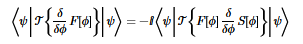$⟨ ψ | 𝒯 { δ δ ϕ F ⁡ [ ϕ ] } | ψ ⟩ = − ⅈ ⁢ ⟨ ψ | 𝒯 { F ⁡ [ ϕ ] ⁢ δ δ ϕ S ⁡ [ ϕ ] } | ψ ⟩$
Differentiable
Manifold
(tangent vector)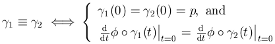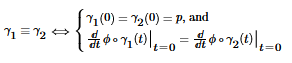$γ 1 ≡ γ 2 ⟺ { γ 1 ⁡ ( 0 ) = γ 2 ⁡ ( 0 ) = p , and ⅆ ⅆ t ⁡ ϕ ∘ γ 1 ⁡ ( t ) | t = 0 = ⅆ ⅆ t ⁡ ϕ ∘ γ 2 ⁡ ( t ) | t = 0$
Cichoń's
Diagram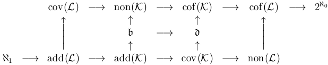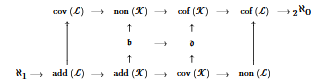$cov ( ℒ ) ⟶ non ( 𝒦 ) ⟶ cof ( 𝒦 ) ⟶ cof ( ℒ ) ⟶ 2 ℵ 0 ↑ ↑ ↑ ↑ 𝔟 ⟶ 𝔡 ↑ ↑ ℵ 1 ⟶ add ( ℒ ) ⟶ add ( 𝒦 ) ⟶ cov ( 𝒦 ) ⟶ non ( ℒ )$
multiscripts
&
greek alphabet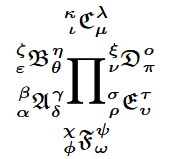$∏ 𝔈 υ τ ρ σ 𝔇 π ο ν ξ 𝔄 δ γ α β 𝔅 θ η ε ζ 𝔉 ω ψ ϕ χ ℭ μ λ ι κ$
nested roots$1 + 2 + 3 + 4 + 5 + 6 + 7 + A 19 17 13 11 7 5 3 ⅇ π = x ‴$
nested
matrices$( ( a 1 a 2 a 3 a 4 a 5 a 6 a 7 a 8 ) ( b 1 b 2 b 3 b 4 ) 0 ( c 1 c 2 c 3 c 4 ) )$
font sizes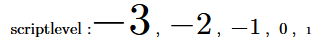## NOTES:

I hope this site can be used as a learning aid (tutorial by example) for mathematics in TeX/LaTeX and in coding MathML.
A small sample of many different types of mathematical expressions and equations is shown.
All the examples are complete with the source code available. (Just click on the equation/formula.)

This web page was validated as:

### Lessons Learned Working on MathML with STIX Fonts on Firefox:

When using an `mtable`, the table cell (`mtd`) default vertical padding produces excessive spacing. Setting the top and bottom padding to zero "`0`" fixes this.

When using the vertical bar "|" (`&vert;`) or integrals, adding a little spacing between themselves and their limits improves the readability of the result.